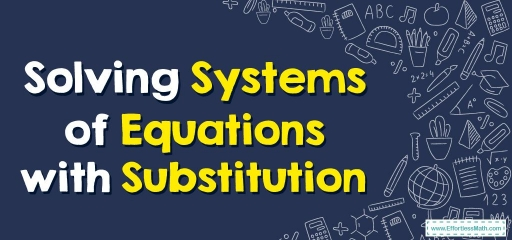# How to Solve Systems of Equations with Substitution?

One of the methods to solve a system of linear equations in two variables is the substitution method. In this post blog, you learn how to solve systems of equations with substitution.we find the value of any one of the variables by isolating it on one side and taking every other term on the other side of the equation. Then we replace that value in the second equation.

## A step-by-step guide to solving systems of equations with substitution

The substitution method is a simple way to solve linear equations and find the solutions to the variables. As the name suggests, it involves finding the value of the $$x$$-variable in terms of the $$y$$-variable and then substituting the value of the $$x$$-variable in the second equation. This way we can solve and find the value of the $$y$$-variable. Finally, we can put the value of $$y$$ in each of the given equations to find $$x$$.

The steps for using the substitution method are as follows:

Let us assume two linear equations: $$2x+3(y+5)=0$$ and $$x+4y+2=0$$.

Step 1: Simplify the given equation by extending the parentheses as needed. So, here we can simplify the first equation to get $$2x+3y+15=0$$.

Now we have two equations,

$$2x + 3y + 15 = 0$$ , equation $$1$$

$$x+4y+2=0$$, equation $$2$$

Step 2: Solve any one of the equations for any one of the variables. You can use any variable based on ease of calculation. Suppose we solve equation $$2$$ for $$x$$. So, we get $$x=-4y-2$$.

Step 3: Substitute the value obtained from $$x$$ into another equation. So we are substituting $$x=-4y-2$$ in the equation $$2x+3y+15=0$$, we get, $$2(-4y-2)+3y+15=0$$.

Step 4: Now simplify the new equation obtained using arithmetic operations. We get, $$-8y-4+3y+15=0$$

$$-5y+11=0$$

$$-5y=-11$$

$$y=\frac{11}{5}$$

Step 5: Now replace the value of $$y$$ in each of the given equations. Let us substitute the value of $$y$$ in equation $$(2)$$.

$$x+4y+2=0$$

$$x+4×(\frac{11}{5})+2=0$$

$$x+ \frac{44}{5}+2=0$$

$$x+ \frac{54}{5}=0$$

$$x=-\frac{54}{5}$$

Therefore, after solving the given linear equations by substitution method, we get $$x= -\frac{54}{5}$$ and $$y=\frac{11}{5}$$.

### Solving Systems of Equations with Substitution – Example 1:

Solve the given system of linear equations by substitution method.

$$-2x – 5 + 3x + y = 0$$, $$3x + y = 11$$

Solution:

As we can see, the first equation can be further simplified by adding $$- 2x$$ and $$3x$$. After simplifying it, we get $$x+y-5=0$$. From this equation, find the value of $$x$$ in terms of $$y$$, which is $$x = 5-y$$. Now substitute this value in equation $$2$$, we get:

$$3(5-y)+y=11$$

$$15-3y+y=11$$

$$15-2y=11$$

$$15-11=2y$$

$$4=2y$$

$$y=2$$

Now, substitute the value of $$y$$ in equation $$1$$. We get, $$x+2-5=0$$, which can be simplified to $$x = 3$$.

## Exercises for Solving Systems of Equations with Substitution

### Solve the given system of linear equations by substitution method.

1. $$\color{blue}{4x+3y = 11 , 3x+4y= 10}$$
2. $$\color{blue}{3x-y=-9 , 2x+4y=8}$$
3.  $$\color{blue}{3x+2y=21 , 4x+5y=21}$$
4. $$\color{blue}{4x-5y=23, 2x+y=-1}$$
1. $$\color{blue}{x=2, y=1}$$
2. $$\color{blue}{x=-2, y=3}$$
3. $$\color{blue}{x=9, y=-3}$$
4. $$\color{blue}{x=\frac{9}{7}, y=-\frac{25}{7}}$$

### What people say about "How to Solve Systems of Equations with Substitution? - Effortless Math: We Help Students Learn to LOVE Mathematics"?

No one replied yet.

X
30% OFF

Limited time only!

Save Over 30%

SAVE $5 It was$16.99 now it is \$11.99